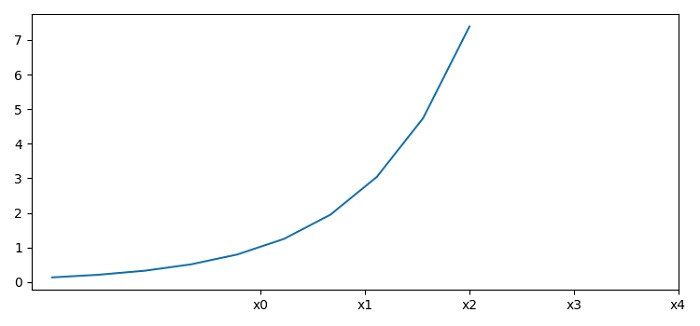How do I convert (or scale) axis values and redefine the tick frequency in Matplotlib?

MatplotlibPythonData Visualization

To convert or scale the axis values and redefine the tick frequency in matplotlib, we can make a list of xticks and xtick_labels using xticks() method. Place the axis scale and redefine the tick frequency.

Steps

• Set the figure size and adjust the padding between and around the subplots.

• Initialize a variable, n, for the number of data points.

• Create x and y data points using numpy.

• Plot x and y data points using plot() method.

• Make lists of ticks and tick labels.

• Use xticks() method to place axis scale and redefine tick frequency.

• To display the figure, use show() method.

Example

import numpy as np
from matplotlib import pyplot as plt
plt.rcParams["figure.figsize"] = [7.50, 3.50]
plt.rcParams["figure.autolayout"] = True
n = 10
x = np.linspace(-2, 2, n)
y = np.exp(x)
plt.plot(x, y)
xticks = [i for i in range(int(n/2))]
xtick_labels = ["x"+str(i) for i in range(int(n/2))]
plt.xticks(xticks, xtick_labels)
plt.show()

Output